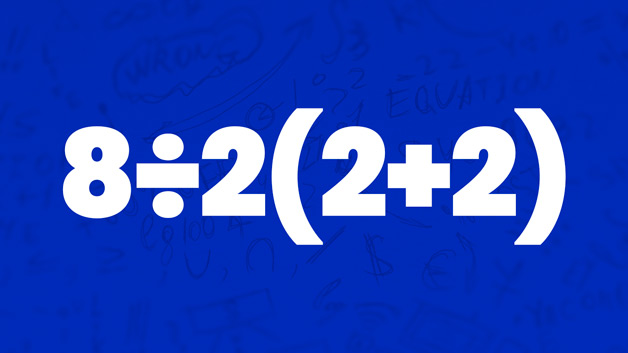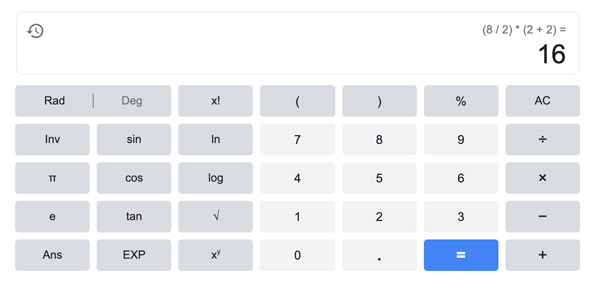# Can You Solve This Viral Maths Question That's Dividing the Internet?This maths equation has been ‘dividing' the internet for years and has many arguing, so what is the actual answer?

The mathematic problem in question is "8÷2 (2+2) = ?” Depending on who you are, there appears to be two answers that most people are arguing over.Half say the answer is 8÷2(2+2) = 1 and the other half say the answer is 8÷2(2+2) = 16. But that depends on what method you use to solve the question.

The answer will depend on which order of operations is followed, whether it be PEMDAS or BODMAS. Both methods agree that the sum within the parentheses or brackets should be solved first, and in this case, it’s 2+2=4.

PEMDAS, which stands for Parentheses, Exponents, Multiplication/Division, Addition/Subtraction is most commonly used in the United States. Using this method, and knowing the first sum is 4, you would solve the sum with the below formula:
8÷(2(4))
= 8÷8
= 1

BODMAS which stands for Brackets, Order, Division/Multiplication, Addition/Subtraction, is most commonly used in Australia and the UK. So for this equation, the process would be: 8÷2×4
= 4×4
= 16

Now, we’re not mathematicians here but if you type the sum in Google, it will give you 16.One mathemetician however, has done a full analysis on the question and he says the answer is also 16. You can read his article below: